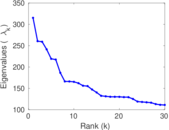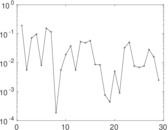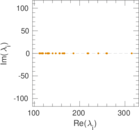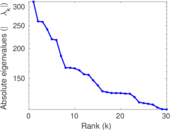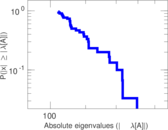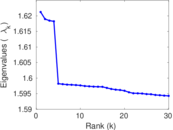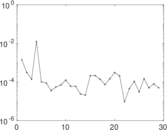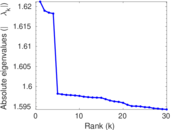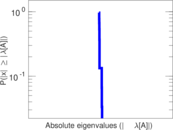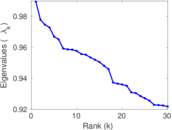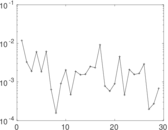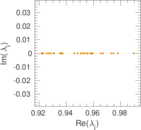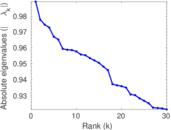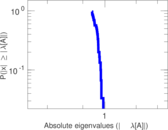This network consists of the wikilinks of the Wikipedia in the Macedonian language (mk). Nodes are Wikipedia articles, and directed edges are wikilinks, i.e., hyperlinks within one wiki. In the wiki source, these are indicated with [[double brackets]]. Only pages in the article namespace are included.

 Code `Wmk` Internal name `wikipedia_link_mk` Name Wikipedia links (mk) Data source http://dumps.wikimedia.org/ AvailabilityDataset is available for download Consistency checkDataset passed all tests Category Hyperlink network Node meaning Article Edge meaning Wikilink Network format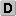Unipartite, directed Edge typeUnweighted, no multiple edges ReciprocalContains reciprocal edges Directed cyclesContains directed cycles Loops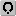Contains loops

## Statistics

 Size n = 136,857 Volume m = 4,903,213 Wedge count s = 3,174,352,469 Claw count z = 7,975,977,540,936 Triangle count t = 90,030,780 Square count q = 29,556,333,984 Maximum degree dmax = 17,475 Maximum outdegree d+max = 3,612 Maximum indegree d−max = 17,466 Average degree d = 71.654 5 Size of LCC N = 136,738 Size of LSCC Ns = 101,648 Relative size of LSCC Nrs = 0.742 731 Diameter δ = 11 50-Percentile effective diameter δ0.5 = 2.861 89 90-Percentile effective diameter δ0.9 = 3.940 20 Median distance δM = 3 Mean distance δm = 3.434 88 Balanced inequality ratio P = 0.217 719 Outdegree balanced inequality ratio P+ = 0.238 062 Indegree balanced inequality ratio P− = 0.204 741 Tail power law exponent with p γ3 = 2.001 00 p-value p = 0.000 00 Outdegree tail power law exponent with p γ3,o = 1.981 00 Outdegree p-value po = 0.000 00 Indegree tail power law exponent with p γ3,i = 1.351 00 Indegree p-value pi = 0.000 00 Degree assortativity ρ = −0.110 606 Degree assortativity p-value pρ = 0.000 00 In/outdegree correlation ρ± = +0.696 288 Clustering coefficient c = 0.085 085 8 Directed clustering coefficient c± = 0.624 295 Operator 2-norm ν = 439.912 Cyclic eigenvalue π = 314.168 Reciprocity y = 0.531 225 Non-bipartivity bA = 0.347 315 Normalized non-bipartivity bN = 0.038 918 1 Algebraic non-bipartivity χ = 0.076 659 3 Spectral bipartite frustration bK = 0.000 363 855

## Plots

### Fruchterman–Reingold graph drawing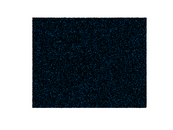### Degree distribution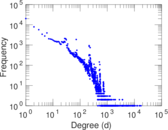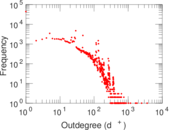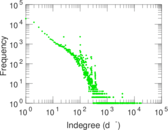### Cumulative degree distribution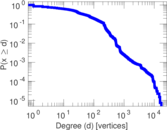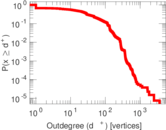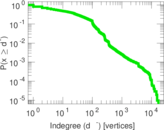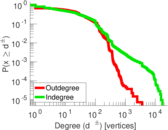### Lorenz curve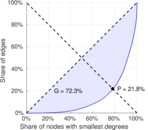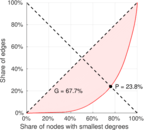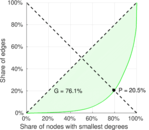### Degree assortativity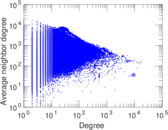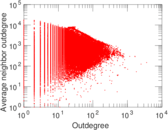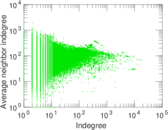### Zipf plot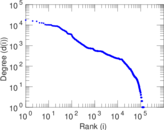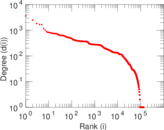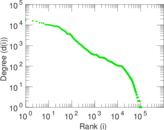### Hop distribution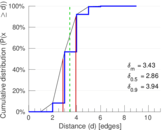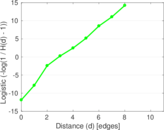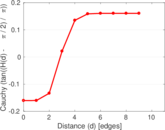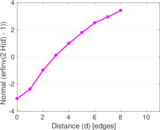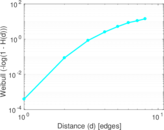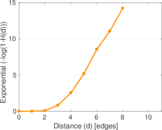### Delaunay graph drawing### In/outdegree scatter plot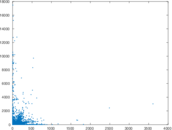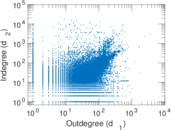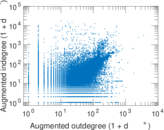### Clustering coefficient distribution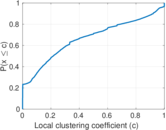### SynGraphy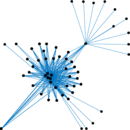### Matrix decompositions plots